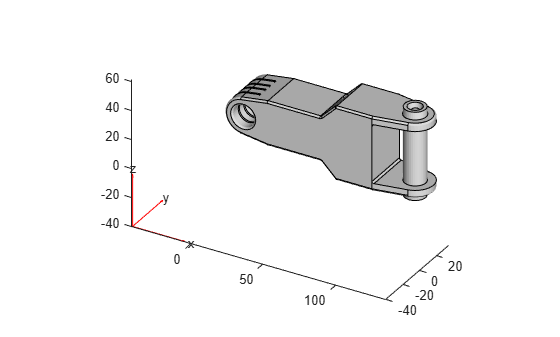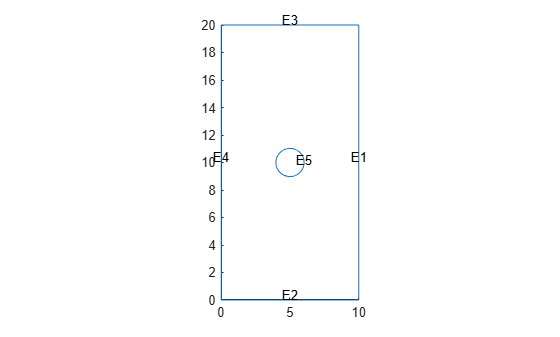importGeometry

Import 2-D or 3-D geometry from STL data

Description

example

gm = importGeometry(geometryfile) creates a geometry object from the specified STL geometry file.

example

importGeometry(model,geometryfile) creates a geometry object from the specified STL geometry file and includes the geometry in the model container.

gm = importGeometry(model,geometryfile) includes the geometry in the model container and also returns the geometry gm to the MATLAB® workspace.

Examples

collapse all

Create a geometry object from an STL geometry file.

Plot the geometry.

pdegplot(gm)Import a planar STL geometry and include it in a PDE model. When importing a planar geometry, importGeometry converts it to a 2-D geometry by mapping it to the X-Y plane.

Create a PDEModel container.

model = createpde;

Import a geometry into the container.

importGeometry(model,'PlateHolePlanar.stl')
ans =
DiscreteGeometry with properties:

NumCells: 0
NumFaces: 1
NumEdges: 5
NumVertices: 5
Vertices: [5x3 double]

Plot the geometry with the edge labels.

pdegplot(model,'EdgeLabels','on')Input Arguments

collapse all

Model object, specified as a PDEModel object, ThermalModel object, StructuralModel object, or ElectromagneticModel object.

Example: model = createpde(3)

Example: structuralmodel = createpde('structural','static-solid')

Example: emagmodel = createpde('electromagnetic','electrostatic')

Path to STL file, specified as a character vector or a string scalar ending with the file extension '.stl' or '.STL'.

Example: '../geometries/Carburetor.stl'

Data Types: char | string

Output Arguments

collapse all

Geometry, returned as a DiscreteGeometry object. See DiscreteGeometry Properties for details.

Limitations

• importGeometry does not allow you to import a multidomain 2-D or 3-D geometry where subdomains have any common points. If the subdomains of the geometry have common points, the toolbox still treats these subdomains as disconnected, without any common interface between them. Each subdomain has its own mesh.

Because of this limitation, you cannot import nested 3-D geometries directly. As a workaround, you can import a mesh and then create a multidomain geometry from the mesh by using the geometryFromMesh function. See Multidomain Geometry Reconstructed from Mesh.

Tips

• The STL format approximates the boundary of a CAD geometry by a collection of triangles, and the importGeometry function reconstructs the faces and edges from this data. Reconstruction from STL data is not precise and can result in a loss of edges and, therefore, the merging of adjacent faces. Typically, lost edges are the edges between two adjacent faces meeting at a small angle, or smooth edges bounding blend surfaces. Usually, the loss of such edges does not affect the analysis workflow.• Because STL geometries are only approximations of the original CAD geometries, the areas and volumes of the STL and CAD geometries can differ.

Introduced in R2015a

Partial Differential Equation Toolbox DocumentationGet trial now